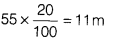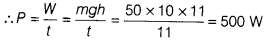# Calculate the work done by Nikhil? Calculate the work done by Nikhil?

Nikhil is a bright student of class VIII. He goes to nearby city stadium every morning for physical exercise with his grandfather. He runs up a staircase of 55 steps in 11s. The height of each step is 20 cm and his weight is 50 kg. [Take, g = 10 ms-2]
(i) Calculate the work done by Nikhil.
(ii) Calculate the work done by Nikhil.

1. Weight of Nikhil = mg = 50 kg x 10 ms-2 = 500 N
Height of the staircase, h =Since, work done = W = mgh ⇒ W = 50 x 10 x 11 = 5500 N
2. Time taken to climb, t = 11 s